# Increase Fluency with Simple Math Dice Games Your Kids Will LOVE

My daughter keeps mixing up her addition facts with her subtraction facts with her multiplication facts. As a fifth grader, she’s responsible for all of it, including division. And her teacher this year is focused on speed along with accuracy.

My daughter has to be able to know 8 + 8 and then 8 x 8, within five seconds of each other.

Knowing math facts is important. But being able to switch between all the math properties is key to problem solving and critical thinking.

It’s also key to solving complex math equations like long division. In one long division problem alone you have to divide, multiply and subtract several times to get to the solution.

Our students’ brains have to be able to switch gears, and switch quickly.

Math dice games will help improve this math skill of being able to transition between adding, subtracting, multiplying and dividing.

Timed test worksheets can get monotonous and boring. Spice things up with math dice games and kids will happily rise to the challenge.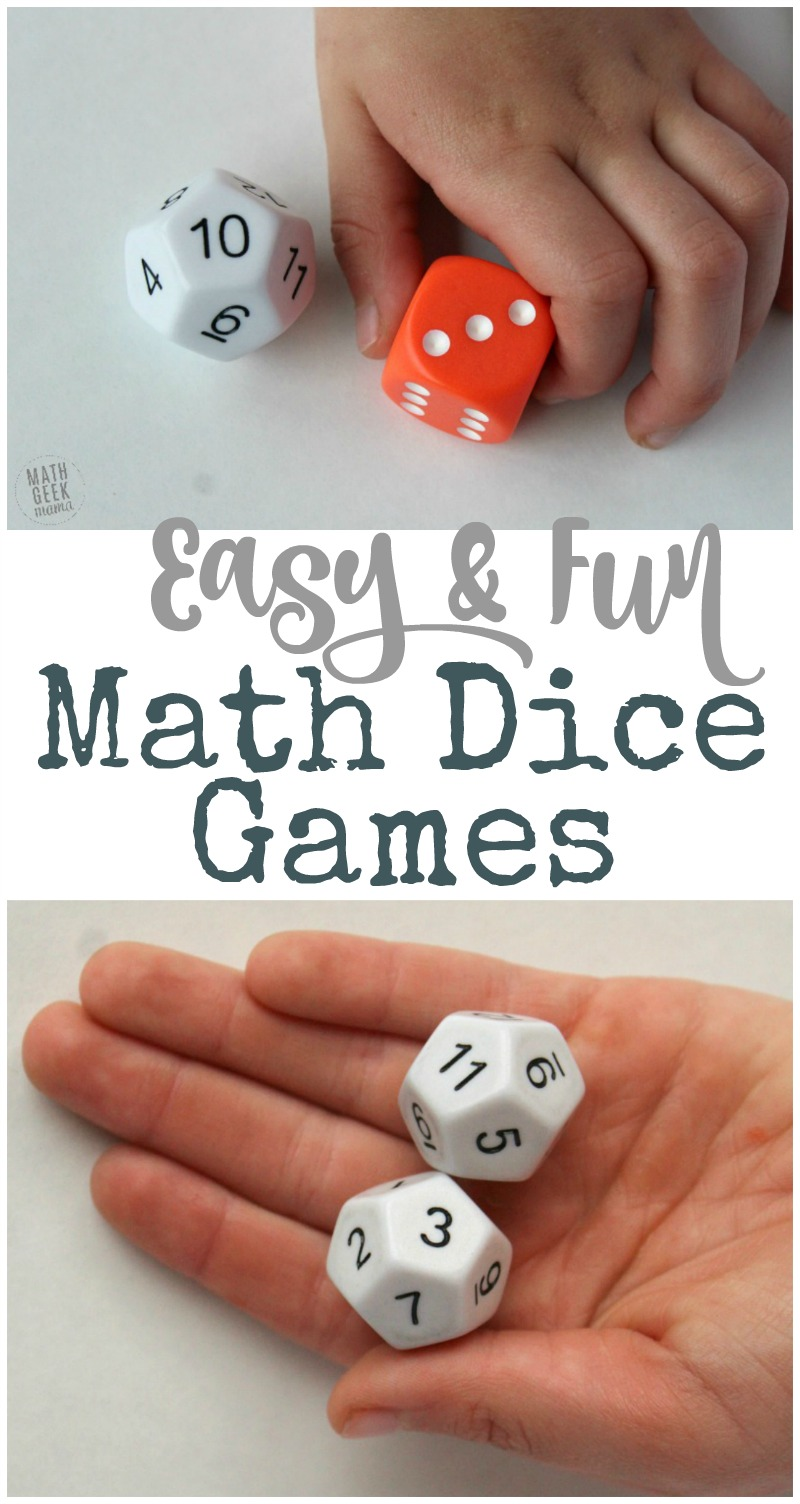*Please Note: This post contains affiliate links which help support the work of this site. Read our full disclosure policy here.*

*This is a guest post from Nicole at Coffee & Carpool.

## Materials Needed for Math Dice Games:

Once a particular dice game level feels “easy” for the student, move them up a level or challenge them to continually improve their math facts and critical thinking skills.

If students need reminders of what math fact to focus on, after they roll you can give them verbal prompts. But the ultimate goal is to help kids feel comfortable and fluent with their math facts.

## Level 1: Add and Subtract up to 6

Use two regular 6-sided dice and roll them. Add them, then subtract them as fast as you can.

For example, with a roll of 5 and 3, my daughter would say “8, 2” then roll again.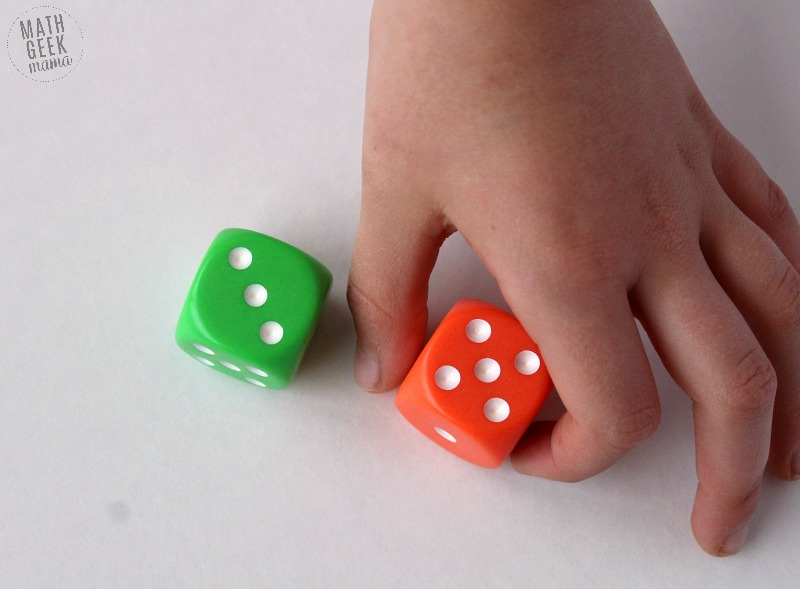## Level 2: Add and Subtract up to 12

Using one 12-sided die and one 6-sided die, add and subtract the numbers as fast as you can.

With a roll of 10 and 3, my daughter would say, “13, 7”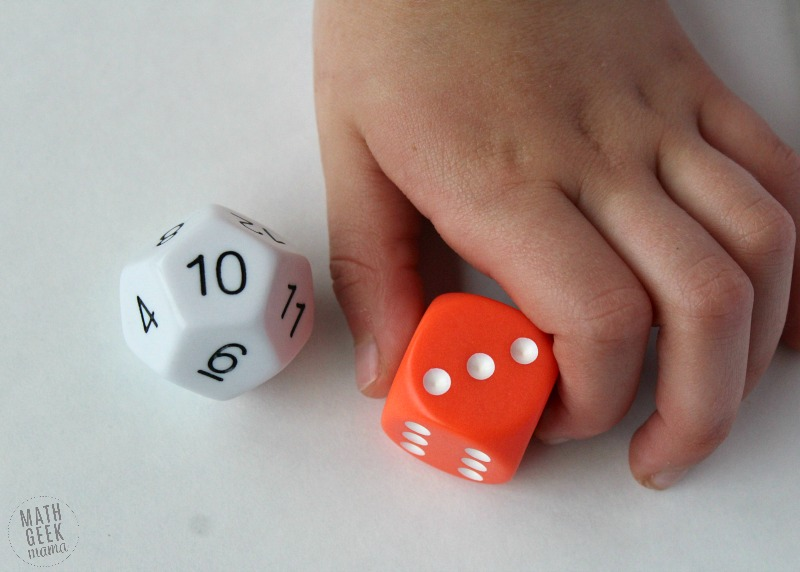## Level 3: Add and Subtract with two numerals up to 12

Using two 12-sided dice, add and subtract the numbers as fast as you can.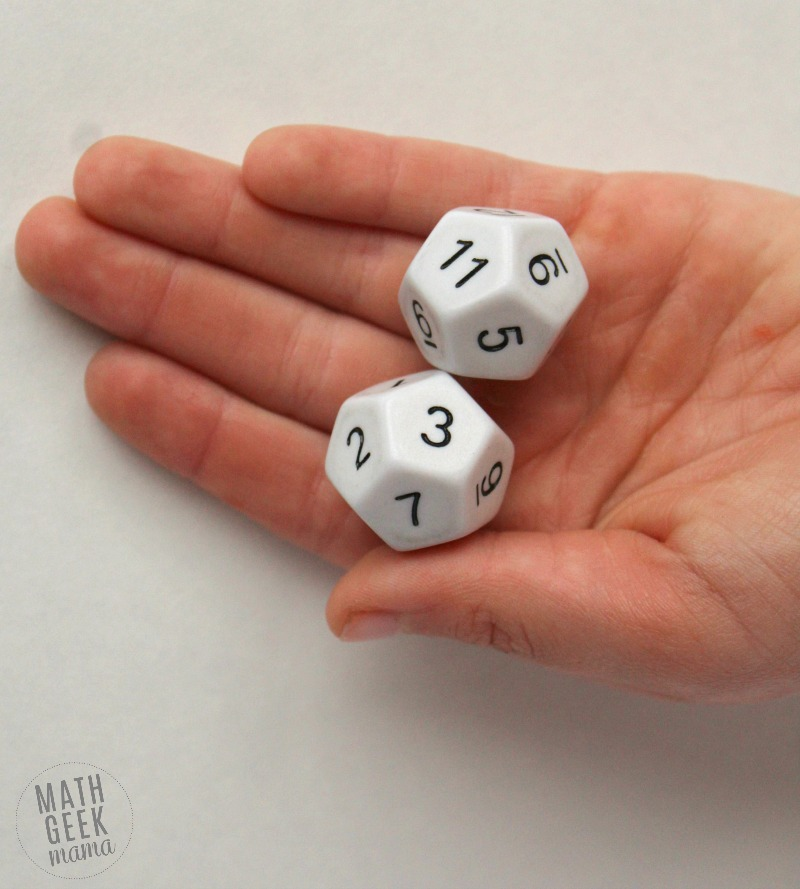For example, with a roll of 9 and 8, my daughter would say, “17, 1”

## Levels 4-6: Adding, Subtracting, Multiplying and Dividing with Remainders Dice Games

Students who have learned multiplication and division can add these math fact skills into the game by starting here at Level 4.

### Level 4: Add, Subtract, Multiply and Divide (when possible) up to 6

Use two regular dice and roll them. Add, subtract, multiply and divide as fast as you can.With a roll of 5 and 3, my daughter would say, “8, 2, 15”

With a roll of 4 and 2, my daughter would say, “6, 2, 8, 2”

### Level 4 Challenge: Add, Subtract, Multiply and Divide using remainders

With a roll of 5 and 3, my daughter would say, “8, 2, 15, 1 R2”

### Level 5: Add, Subtract, Multiply and Divide (when possible) with one factor up to 12

Use one 12-sided die and one 6-sided die to add, subtract, multiply and divide (if possible) as fast as you can.

With a roll of 10 and 3, my daughter would say “13, 7, 30”

With a roll of 10 and 5, my daughter would say “15, 5, 50, 2”

### Level 5 Challenge: Add, Subtract, Multiply and Divide using remainders with one factor up to 12

Use one 12-sided die and one 6-sided die to add, subtract, multiply and divide with a remainder as fast as you can.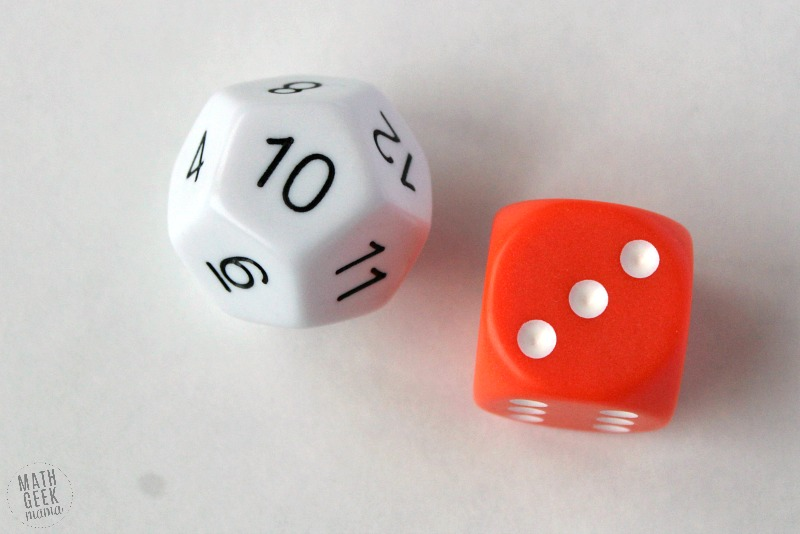With a roll of 10 and 3, my daughter would say “13, 7, 30, 3 R1”

### Level 6: Add, Subtract, Multiply and Divide (when possible) with both factors up to 12

Use two 12-sided dice to add, subtract, multiply and divide (if possible) as fast as you can.

With a roll of 9 and 8, my daughter would say “17, 1, 72”

With a roll of 12 and 12 and my daughter would say “24, 0, 144, 1”

### Level 6 Challenge: Add, Subtract, Multiply and Divide using remainders with both factors up to 12

Use two 12-sided dice to add, subtract, multiply and divide with remainders as fast as you can.

With a roll of 9 and 8, my daughter would say “17, 1, 72, 1 R1”

The faster they know their math facts, the faster they will be able to attack more complex math problems with both confidence and accuracy…which is the ultimate goal for every math student.

Hopefully these math dice games are a fun and helpful way to reach all your kids!

Nicole is a recovering elementary school teacher who uses every trick she knows from her teaching days to get her kids to love math. She writes about Mom Hacks, School Success Tips and Support for Parents on the Go at Coffee and Carpool. You can follow her on Facebook or on Pinterest.

## More Easy Math Games to Increase Fact Fluency: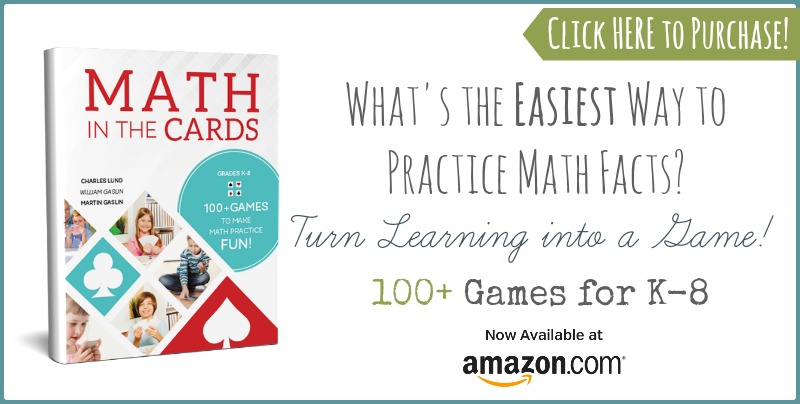### Never Run Out of Fun Math Ideas

If you enjoyed this post, you will love being a part of the Math Geek Mama community! Each week I send an email with fun and engaging math ideas, free resources and special offers. Join 163,000+ readers as we help every child succeed and thrive in math! PLUS, receive my FREE ebook, 5 Math Games You Can Play TODAY, as my gift to you!

## One Comment

1.Stacey says:

I love your 5 simple math games using cards. I played “Spiral” this morning with my 2nd grade class. I was able to have two groups work on their multiplying, one group with 2 dices addition and one group with one die addition. Simple and easy to teach to each group. Thank you